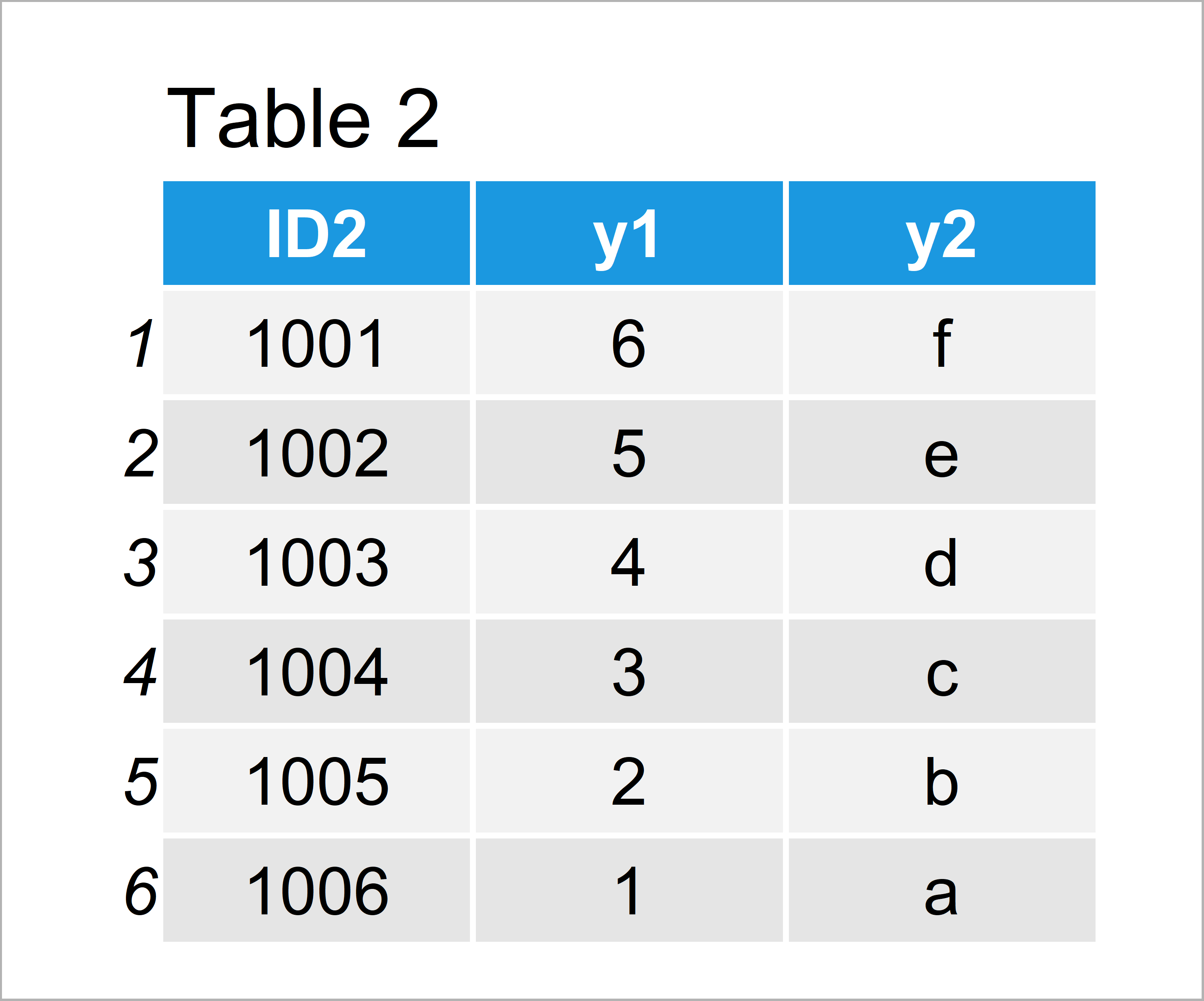# Merge Two data.table Objects in R (Example)

In this tutorial you’ll learn how to combine two data.table objects in the R programming language.

Let’s get started…

## Example Data & Add-On Packages

We first need to install and load the data.table package, to be able to use the corresponding functions:

```install.packages("data.table")          # Install data.table package

Now, we can create two example data.tables:

```data1 <- data.table(ID1 = 1001:1006,    # Create first data.table
x1 = 1:6,
x2 = letters[1:6])
data1                                   # Print first data.table```Table 1 visualizes the output of the RStudio console and shows that our example data.table consists of six rows and three columns.

Let’s create another data.table in R:

```data2 <- data.table(ID2 = 1001:1006,    # Create second data.table
y1 = 6:1,
y2 = letters[6:1])
data2                                   # Print second data.table```By running the previous code we have created Table 2, i.e. a second data.table with different ID column.

## Example: Merge Two data.tables with Different IDs Using merge.data.table Function

This example shows how to join our two data.tables using the merge.data.table function. For this, we have to use the following R code:

```data_all <- merge.data.table(data1,     # Merge data.tables
data2,
by.x = "ID1",
by.y = "ID2")
data_all                                # Print merged data.table```By running the previous code we have created Table 3, i.e. a merged version of our two input data.tables.

Note that we had to specify two different ID columns using the by.x and by.y arguments, since our two input data.tables had different ID column names. In case both data.tables would have the same ID column name, we could simply use the by argument and specify the ID name only once.

## Video & Further Resources

Do you need further information on the R programming codes of this post? Then I can recommend having a look at the following video of my YouTube channel. I’m explaining the content of this tutorial in the video.

Furthermore, you may read the other tutorials on this homepage: